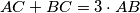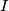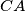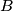### IMO Shortlist 2005 problem G1

Kvaliteta:
Avg: 3.3
Težina:
Avg: 6.0
Given a triangle$ABC$ satisfying$AC+BC=3\cdot AB$. The incircle of triangle$ABC$ has center$I$ and touches the sides$BC$ and$CA$ at the points$D$ and$E$, respectively. Let$K$ and$L$ be the reflections of the points$D$ and$E$ with respect to$I$. Prove that the points$A$,$B$,$K$,$L$ lie on one circle.
Source: Međunarodna matematička olimpijada, shortlist 2005